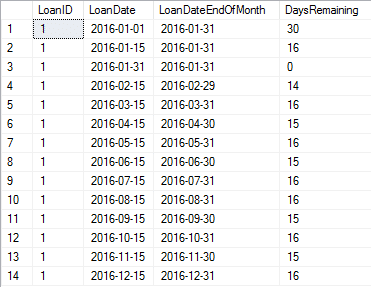# Calculate the Last Day of the Month using SQL

By: Kris Wenzel   |   Updated: March 6, 2022
Works With: SQL How To

When working with SQL dates, sometimes you need to calculate the end of the month.  Months are tricky!  Some are 28 days, others 30 or 31, and now and then there’s a leap year!

So, given a date, how do you calculate the number of days remaining in the month?

The calculation is really a two-step process:

1. Determine the last date of the month.
2. Calculate the difference in days, between the date in question and the step 1.

We can use the DATEDIFF function do calculate the difference in days, but how do you calculate last day of the month?

## Using EOMONTH to Calculate the End of the Month

In SQL Server 2012 and above, you can use the EOMONTH function to return the last day of the month.

For example

`SELECT EOMONTH('02/04/2016')`

Returns  02/29/2016

As you can see the EOMONTH function takes into account leap year.

So to calculate the number of day from a date to the end of the month you could write

`SELECT DATEDIFF(d,'02/04/2016', EOMONTH('02/04/2016'))`

Which returns 25.

Let’s try a more comprehensive example that calculates the days remaining on a LoanDate for the LoanDate’s current month:

```BEGIN TRANSACTION
-- Sample Data
CREATE TABLE LoanDate (LoanID INT, LoanDate DATE);
INSERT INTO LoanDate Values (1, '1/1/2016');
INSERT INTO LoanDate Values (1, '1/15/2016');
INSERT INTO LoanDate Values (1, '1/31/2016');
INSERT INTO LoanDate Values (1, '2/15/2016');
INSERT INTO LoanDate Values (1, '3/15/2016');
INSERT INTO LoanDate Values (1, '4/15/2016');
INSERT INTO LoanDate Values (1, '5/15/2016');
INSERT INTO LoanDate Values (1, '6/15/2016');
INSERT INTO LoanDate Values (1, '7/15/2016');
INSERT INTO LoanDate Values (1, '8/15/2016');
INSERT INTO LoanDate Values (1, '9/15/2016');
INSERT INTO LoanDate Values (1, '10/15/2016');
INSERT INTO LoanDate Values (1, '11/15/2016');
INSERT INTO LoanDate Values (1, '12/15/2016');

-- Select LoanDate, Days in Month, and Days Remaining in Month
SELECT LoanID,
LoanDate,
EOMONTH(LoanDate) LoanDateEndOfMonth,
DATEDIFF(d, LoanDate, EOMONTH(LoanDate)) as DaysRemaining
FROM   LoanDate
ROLLBACK```

Here is the result:As you can see, the DaysRemaining changes according to the month.  Also, notice that when the date occurs on the same date as the end of the month, such as row 3, zero days remain.

Now let’s look at how you would go about calculating this if you are using SQL 2008 R2 or before.

## Old School Method to Calculate Last Day of Month

The calculation is really the same two-step process:

1. Determine the last date of the month
2. Calculate the difference in days, between the date in question and the step 1.

However, the difference is in how we determine the last date of the month.  Since EOMONTH isn’t available, we need to calculate it the “old fashioned” way.  There are several methods to do this.  Here is one.

We’ll calculate the last day of the month using two functions:  DATEADD and DAY.

We’ll use DATEADD to add a month to the date.  Then the DAY function to determine the number of days from the beginning of the month.  By subtracting this from the date we just calculated (the one that is a month ahead), we can get the last date of the month.

OK, I know that sounds confusing, so let’s break it down using this diagram:Using the same example as we did with EOMONTH we have the following statement we can use to test:

```BEGIN TRANSACTION
-- Sample Data
CREATE TABLE LoanDate (LoanID INT, LoanDate DATE);
INSERT INTO LoanDate Values (1, '1/1/2016');
INSERT INTO LoanDate Values (1, '1/15/2016');
INSERT INTO LoanDate Values (1, '1/31/2016');
INSERT INTO LoanDate Values (1, '2/15/2016');
INSERT INTO LoanDate Values (1, '3/15/2016');
INSERT INTO LoanDate Values (1, '4/15/2016');
INSERT INTO LoanDate Values (1, '5/15/2016');
INSERT INTO LoanDate Values (1, '6/15/2016');
INSERT INTO LoanDate Values (1, '7/15/2016');
INSERT INTO LoanDate Values (1, '8/15/2016');
INSERT INTO LoanDate Values (1, '9/15/2016');
INSERT INTO LoanDate Values (1, '10/15/2016');
INSERT INTO LoanDate Values (1, '11/15/2016');
INSERT INTO LoanDate Values (1, '12/15/2016');

-- Select LoanDate, Days in Month, and Days Remaining in Month
SELECT LoanID,
LoanDate,
FROM   LoanDate
ROLLBACK```

Here are the results:

So which method should you use?

If I’m writing code and I know will run on SQLServer 2012 or greater, I would go for the EOMONTH method as it is much easier to read; however, I think knowing and understanding the method using DATEADD and DAY help you better understand how to manipulate dates.

So what other ways have you see to calculate the last date in a month?  If would love to see them.  Just let me know in the comments below.